Function Repository Resource:

# PetriNetNondeterministicEvolution

Evolve a Petri net configuration nondeterministically by randomly selecting one possible transition firing at each step

Contributed by: Jonathan Gorard
 ResourceFunction["PetriNetNondeterministicEvolution"][pnet,n] evolves a Petri net configuration defined by the PetriNetObject expression pnet nondeterministically for n steps, by randomly selecting one possible transition firing at each step. ResourceFunction["PetriNetNondeterministicEvolution"][p,t,a,init,n] evolves a Petri net defined by the list of places p, the list of transitions t and the list of arcs a, with initial token distribution init, nondeterministically for n steps, by randomly selecting one possible transition firing at each step. ResourceFunction["PetriNetNondeterministicEvolution"][assoc,init,n] evolves a Petri net defined by the association of places, transitions and arcs assoc, with initial token distribution init, nondeterministically for n steps, by randomly selecting one possible transition firing at each step. ResourceFunction["PetriNetNondeterministicEvolution"][…,prop] gives the property "prop" for the specified nondeterministic Petri net evolution.

## Details and Options

A Petri net is an abstract mathematical representation of a distributed system (frequently used for describing chemical reactions, biological processes, concurrent computing, etc.) consisting of a directed bipartite graph whose vertices are classified as either "places" or "transitions". The directed edges connecting places and transitions together are known as "arcs".
Each place can contain a number of "tokens", and a given transition will "fire" if the places connected to it by input arcs all contain at least one token. When a transition fires, it redistributes tokens from places connected to it by input arcs to places connected to it by output arcs.
ResourceFunction["PetriNetNondeterministicEvolution"] supports the specification of Petri nets either by PetriNetObject expressions, a collection of three lists (places p, transitions t and arcs a), or by an explicit association of the form <|"Places"p,"Transitions"t,"Arcs"a|>. The list of arcs a should be a list of either rules or DirectedEdge objects (each connecting a place to a transition, or vice versa).
PetriNetObject expressions can be constructed using the MakePetriNet resource function.
When specifying Petri nets in terms of lists or associations, the list init should be a list of integers specifying how many initial tokens to assign to each place. If a list init is not given, ResourceFunction["PetriNetNondeterministicEvolution"] will assume that each place contains no tokens.
ResourceFunction["PetriNetNondeterministicEvolution"] evolves the specified Petri net nondeterministically by randomly selecting and applying one possible transition firing at each step.
When no step count n is specified, ResourceFunction["PetriNetNondeterministicEvolution"] will evolve the Petri net nondeterministically for a single step and return a single result. When a step count n is specified, ResourceFunction["PetriNetNondeterministicEvolution"] will evolve the Petri net nondeterministically for n steps and return a list of the intermediate results at each step.
When no property prop is specified, ResourceFunction["PetriNetNondeterministicEvolution"] will assume the "PetriNetObjects" property (i.e. it will return a list of the PetriNetObject expressions obtained during the evolution).
In ResourceFunction["PetriNetNondeterministicEvolution"][,"prop"], the following properties can be requested:
 "PetriNetObjects" list of PetriNetObject expressions obtained "LabeledGraphs" list of directed graph representations obtained (with token counts represented graphically) "LabeledGraphsHighlighted" list of directed graph representations obtained (with token counts represented graphically), and with transition firings highlighted "WeightedGraphs" list of directed graph representations obtained (with token counts represented as vertex weights) "WeightedGraphsHighlighted" list of directed graph representations obtained (with token counts represented as vertex weights), and with transition firings highlighted "Tokens" list of token numbers associated to each place after each firing "TokenFirings" list of token numbers associated to each place after each firing, with the corresponding transition firing specified
ResourceFunction["PetriNetNondeterministicEvolution"] accepts the same options as Graph.
In a PetriNetObject expression, the following properties are supported:
 "AssociationForm" Petri net represented as an association of places, transitions and arcs "Places" list of places in the Petri net "PlaceCount" number of places in the Petri net "Transitions" list of transitions in the Petri net "TransitionCount" number of transitions in the Petri net "Arcs" list of arcs in the Petri net "ArcCount" number of arcs in the Petri net "Tokens" list of token numbers associated to each place in the Petri net "TokenCount" total number of tokens across all places in the Petri net "UnlabeledGraph" directed graph form of the Petri net without token counts represented graphically "LabeledGraph" directed graph form of the Petri net with token counts represented graphically "WeightedGraph" directed graph form of the Petri net with token counts represented as vertex weights
By convention, ResourceFunction["PetriNetNondeterministicEvolution"] renders places as circles, transitions as squares, arcs as directed edges and tokens as black dots.

## Examples

### Basic Examples (3)

Construct a simple Petri net from lists of four places, three transitions and eight arcs, with an initial distribution of six tokens:

 In:=Out=Evaluate one step in the nondeterministic evolution of this Petri net:

 In:=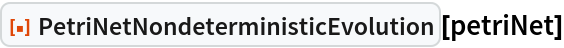Out=Evaluate the first four steps in the nondeterministic evolution of this Petri net:

 In:=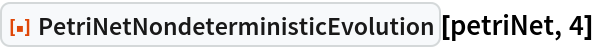Out=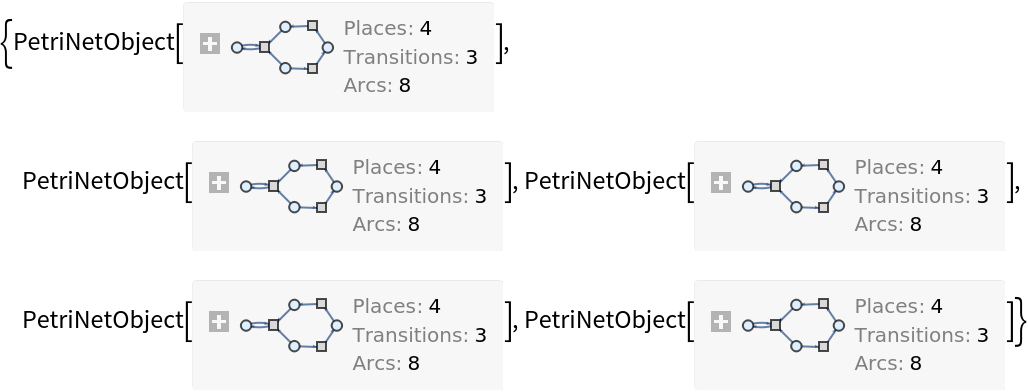Show the labeled graph obtained after one step of nondeterministic evolution, with the random transition firing highlighted:

 In:=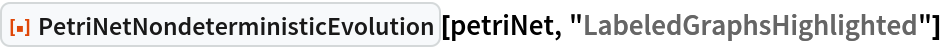Out=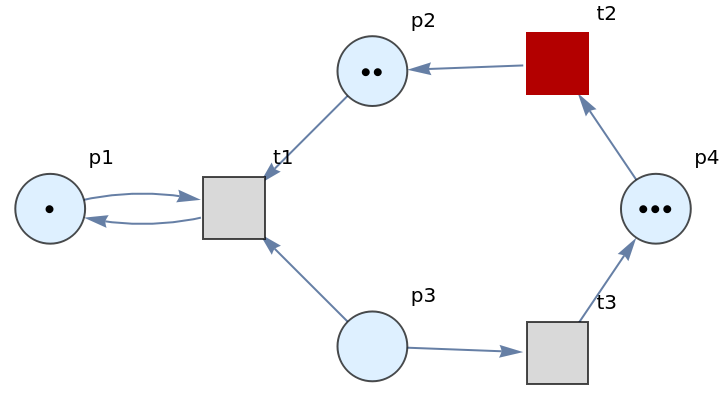Show the labeled graph obtained after one step of nondeterministic evolution, but without any transition firing highlighted:

 In:=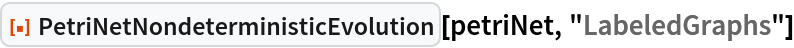Out=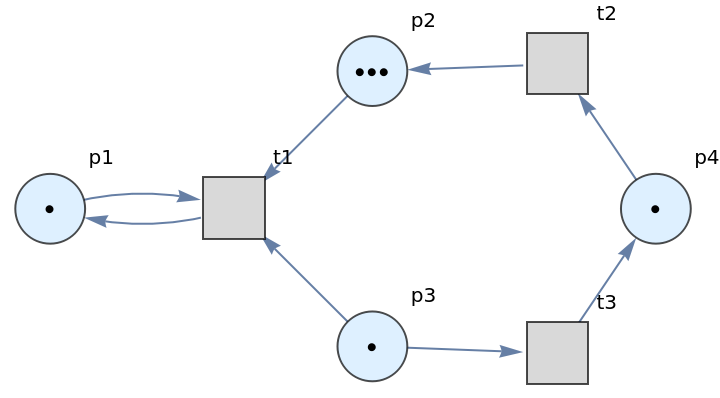Show the labeled graphs obtained after four steps of nondeterministic evolution, with the random transition firings highlighted:

 In:=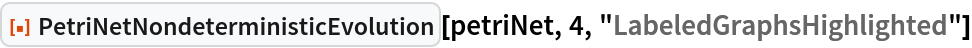Out=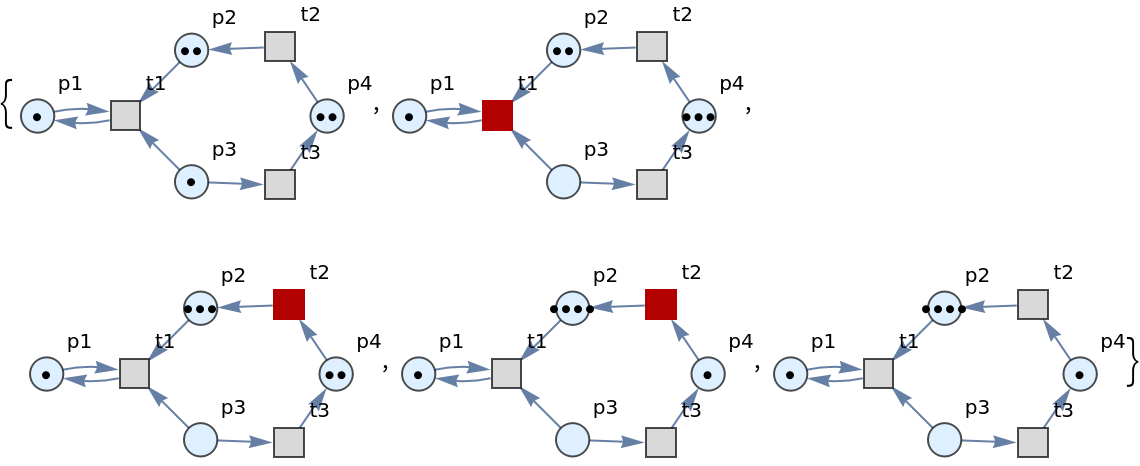Evolve a Petri net (representing a reversible chemical reaction) specified by an association of three places, two transitions and six arcs, with an initial distribution of twelve tokens, for four steps nondeterministically:

 In:=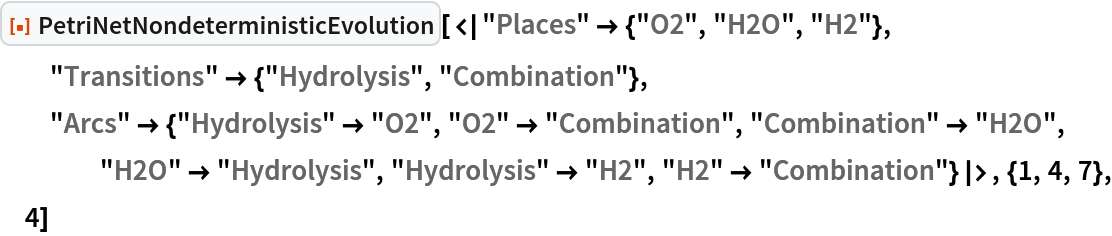Out=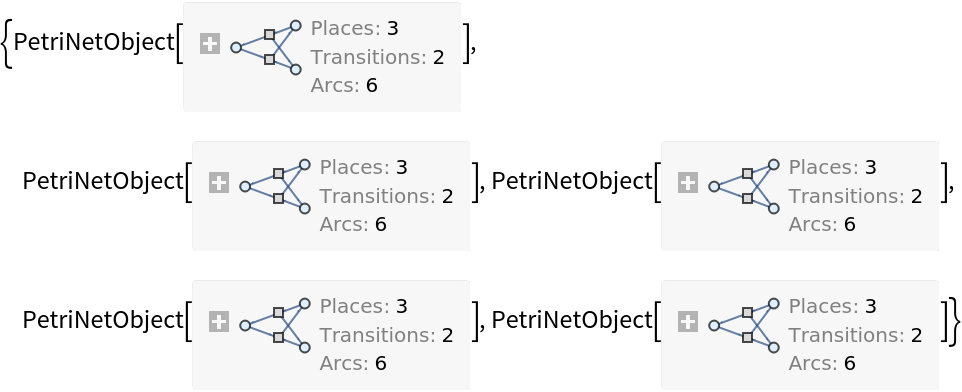Show the list of token numbers obtained after each of the four steps:

 In:=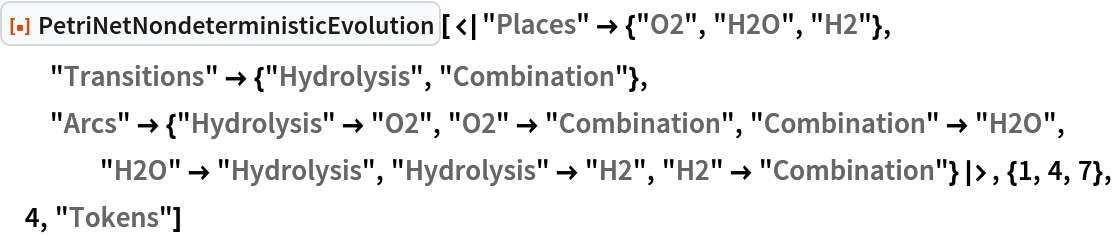Out=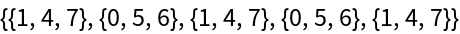Show the list of token numbers obtained after each of the four steps, along with the corresponding random transition firings:

 In:=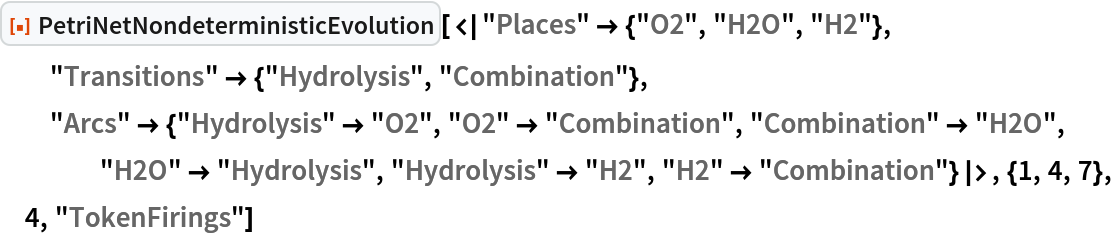Out=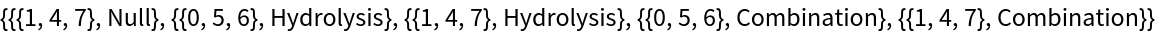Show the labeled graphs obtained after four steps of nondeterministic evolution, with the random transition firings highlighted:

 In:=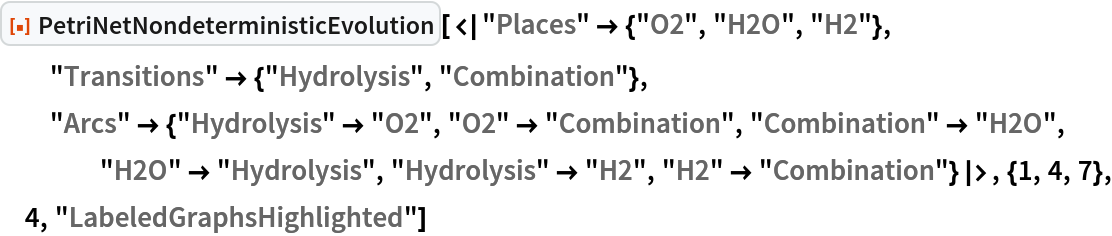Out=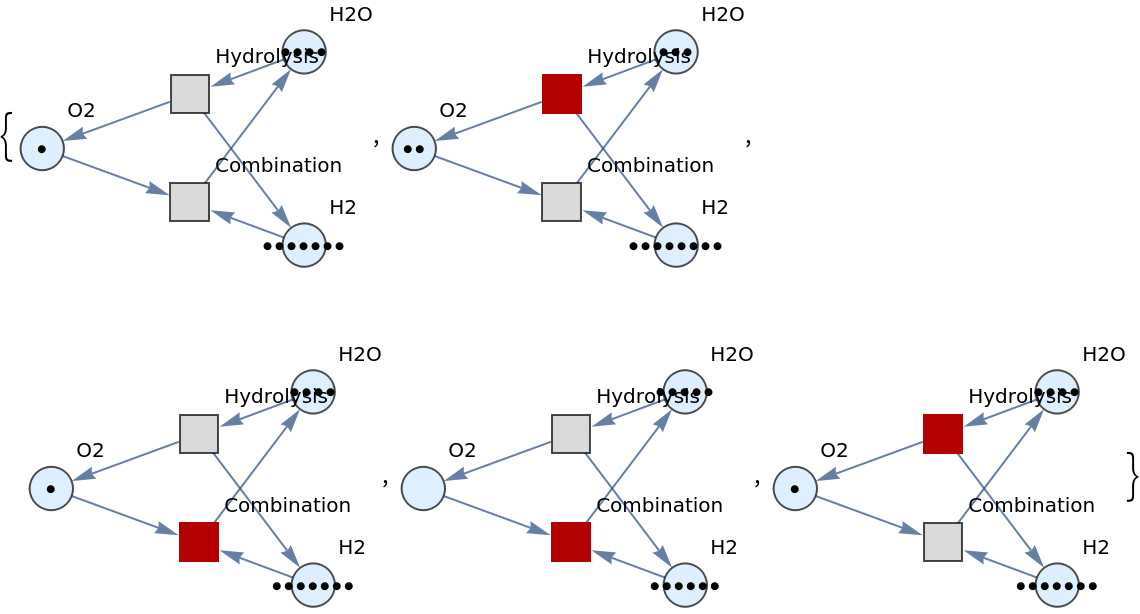Show the weighted graphs obtained after four steps of nondeterministic evolution, with the random transition firings highlighted:

 In:=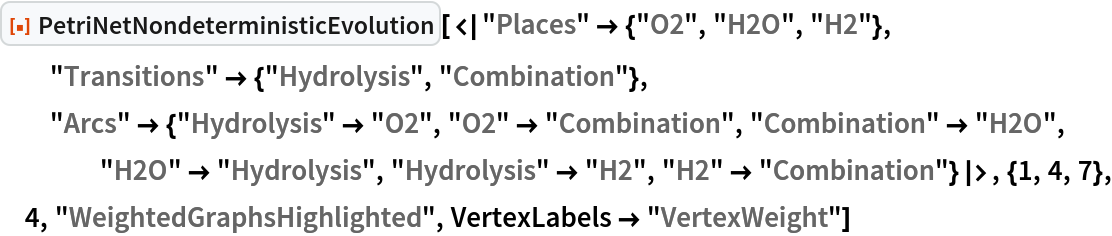Out=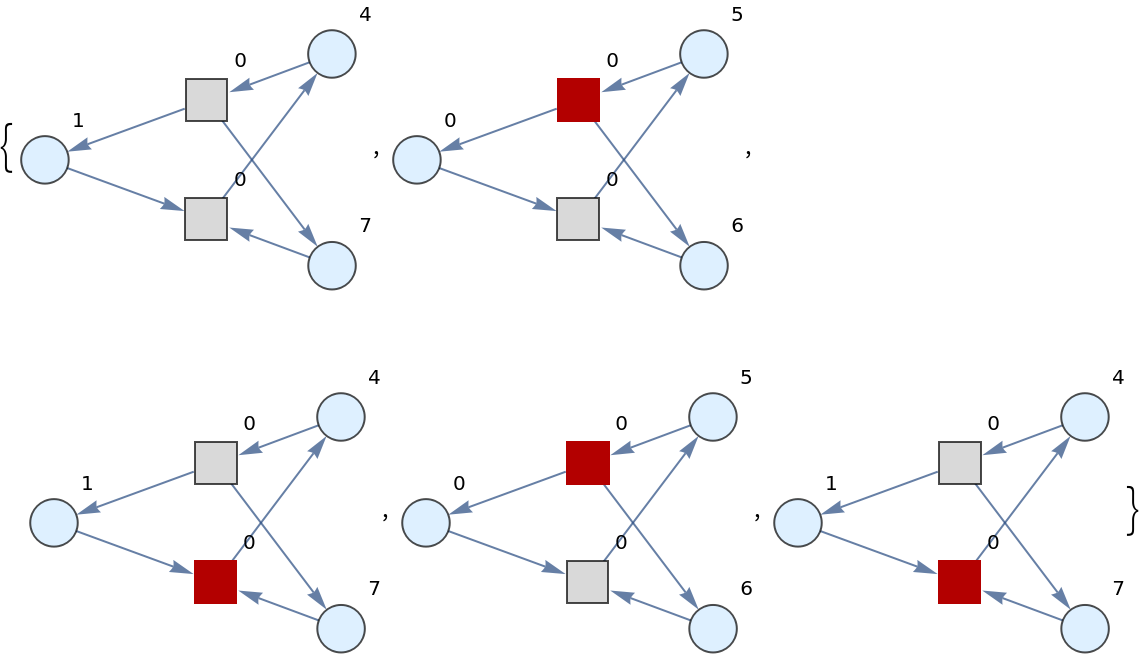Show the weighted graphs obtained after four steps of nondeterministic evolution, but without any transition firings highlighted:

 In:=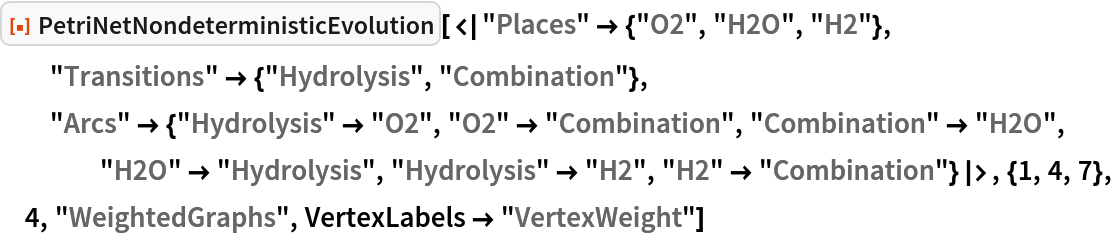Out=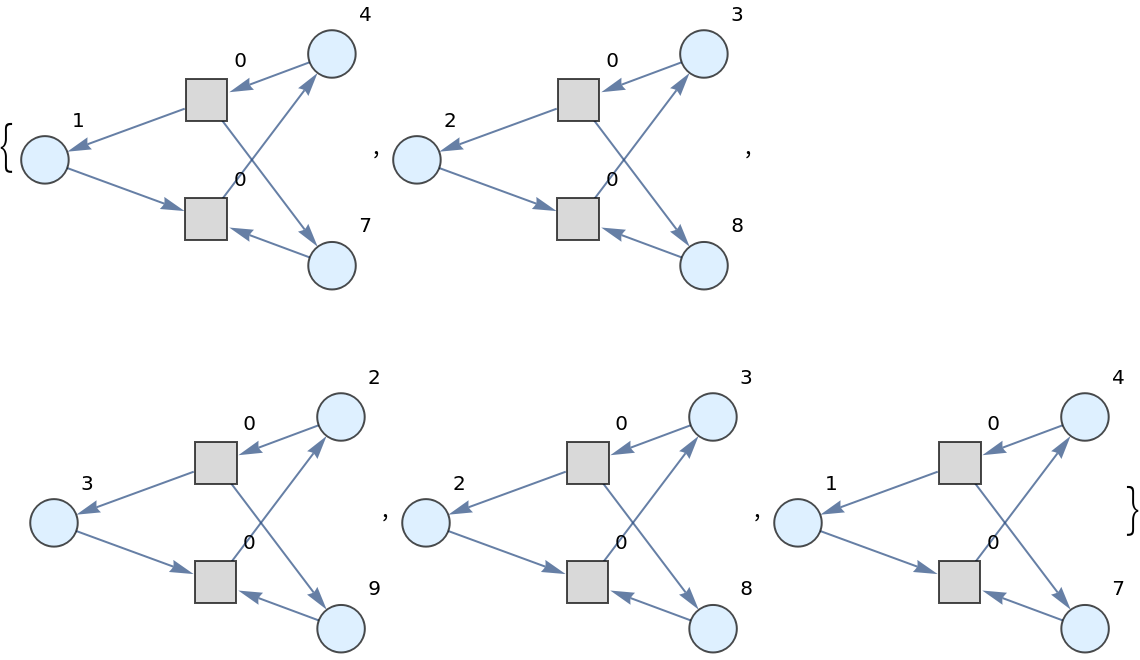Evolve a more complicated Petri net representing a concurrent communications protocol between two agents (specified as lists of places, transitions, arcs and tokens) for four steps nondeterministically:

 In:=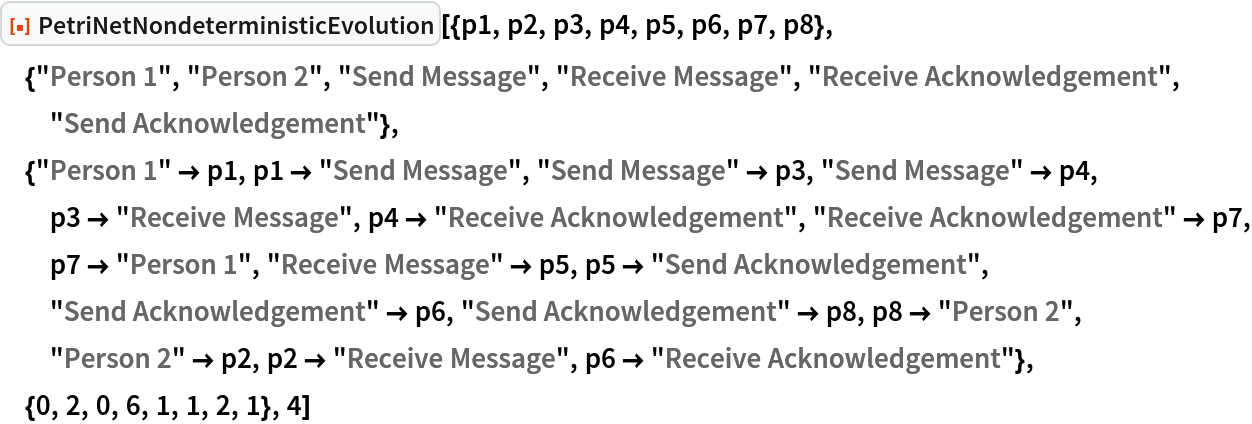Out=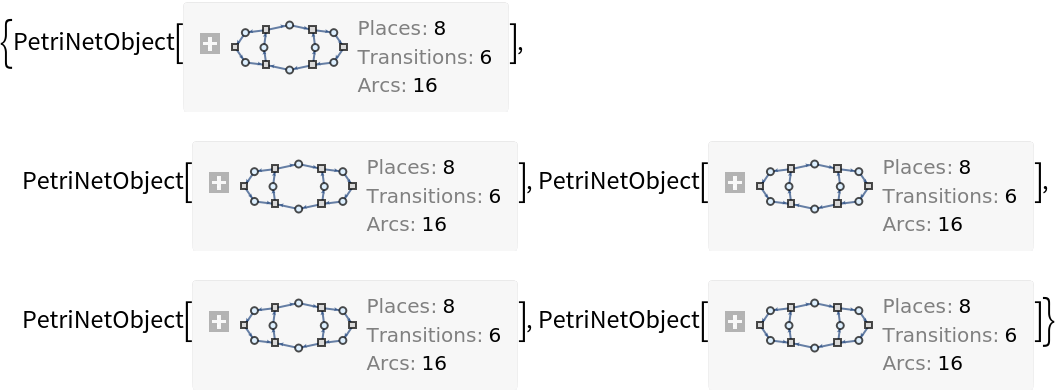Show the list of token numbers obtained after each of the four steps, along with the corresponding random transition firings:

 In:=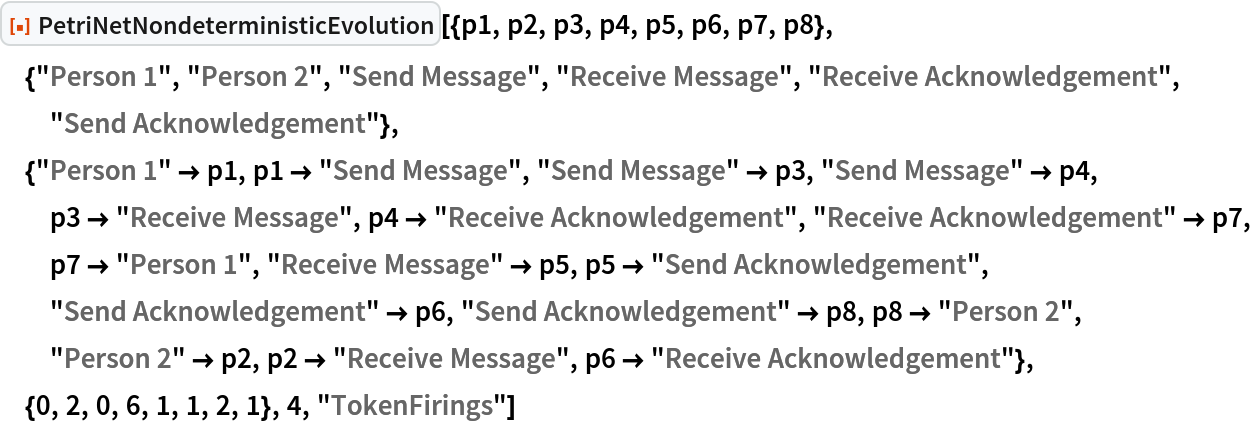Out=### Scope (2)

If PetriNetNondeterministicEvolution is called without an explicit list of token numbers, it is assumed that each place contains no tokens:

 In:=Out=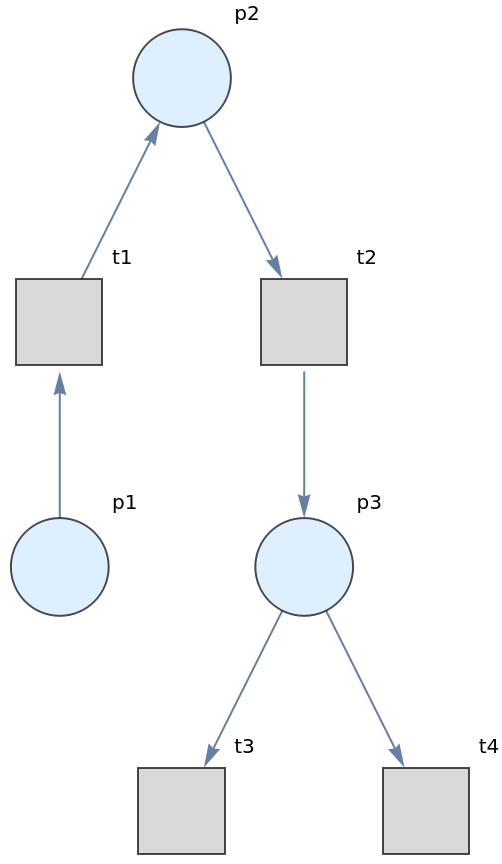Construct a Petri net representing a simple producer-consumer problem in concurrency theory:

 In:=Out=Show the list of Petri net objects obtained after four steps of nondeterministic evolution:

 In:=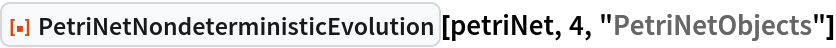Out=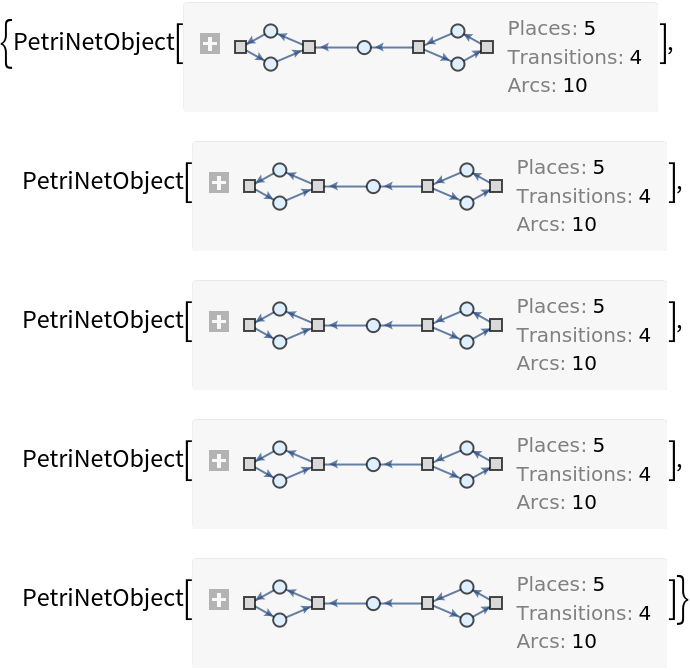Show the list of directed graph representations (with token counts represented graphically) after four steps of nondeterministic evolution:

 In:=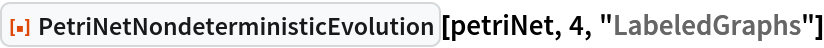Out=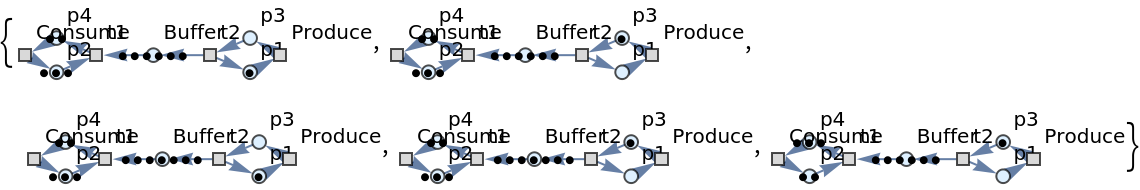Show the list of directed graph representations (with token counts represented graphically, and with transition firings highlighted) after four steps of nondeterministic evolution:

 In:=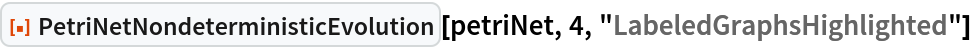Out=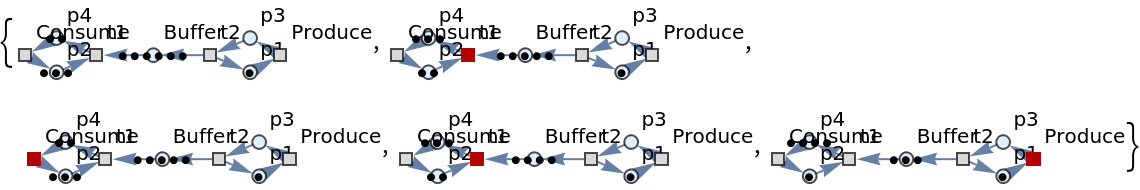Show the list of directed graph representations (with token counts represented as vertex weights) after four steps of nondeterministic evolution:

 In:=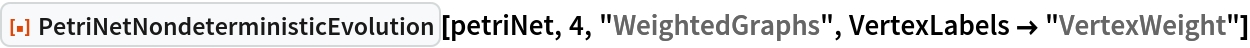Out=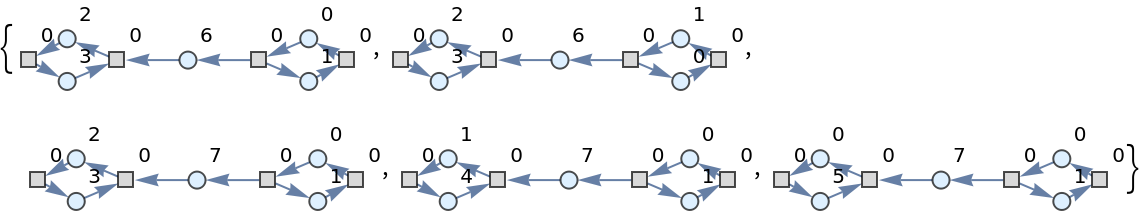Show the list of directed graph representations (with token counts represented as vertex weights, and with transition firings highlighted) after four steps of nondeterministic evolution:

 In:=Out=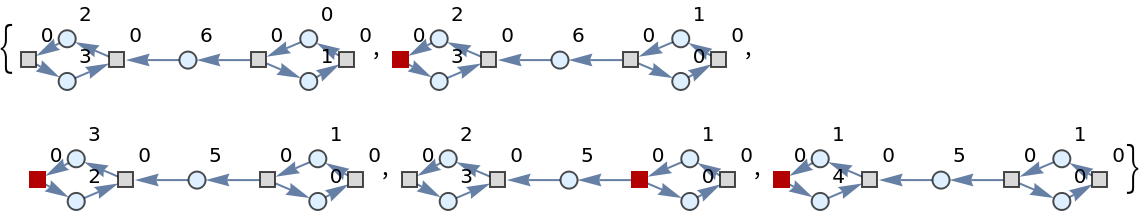Show the list of token numbers associated to each place after each firing, following four steps of nondeterministic evolution:

 In:=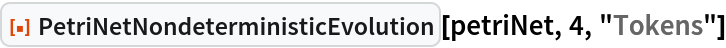Out=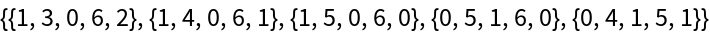Show the list of token numbers associated to each place after each firing (with the corresponding transition firings specified), following four steps of nondeterministic evolution:

 In:=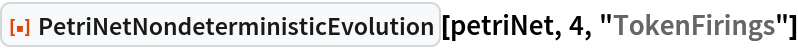Out=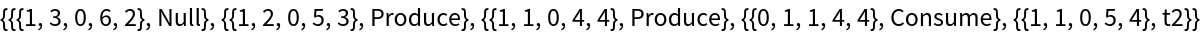### Options (1)

PetriNetNondeterministicEvolution supports the same options as Graph:

 In:=Out=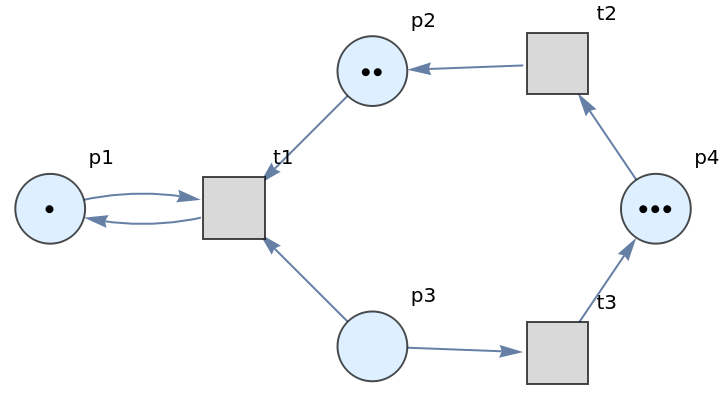In:=Out=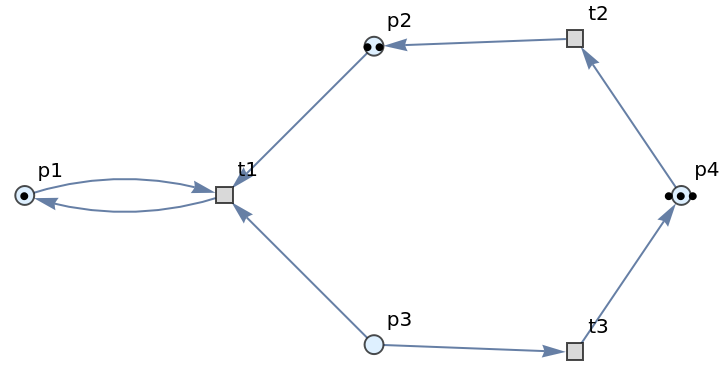Jonathan Gorard

## Version History

• 1.0.0 – 13 December 2021